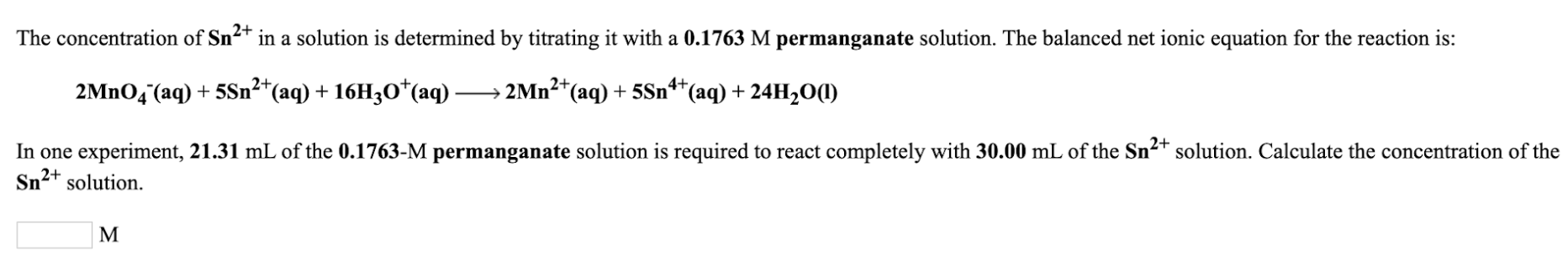# The concentration of Sn^2+ in a solution is determined by titrating it with a 0.1763 M permanganate solution. The balanced net ionic equation for the reaction is: 2MnO4^-(aq) + 5Sn^2+(aq) + 16H3O^+(aq) → 2Mn^2+ (aq) + 5Sn^4+(aq) + 24H2O(l) In one experiment, 21.31 mL of the 0.1763-M permanganate solution is required to react completely with 30.00 mL of the Sn^2+ solution. Calculate the concentration of the Sn^2+ solution.